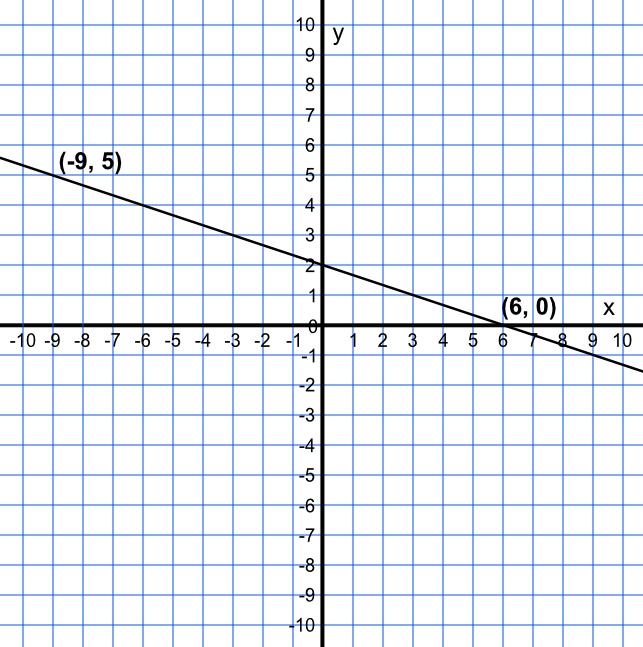Equation of a Line

## Equation of a Line

With two points on a straight line, you can find the equation of a line.

Calculate the gradient from the two coordinates (use frac(up)(along), or the formula m=(y_2-y_1)(x_2-x_1) where (x_1, y_1) and (x_2, y_2) are the coordinates). This gives a value for the gradient, m.

Substitute the value for m, and the values from one of the coordinates (it does not matter which one) into y = mx + c to obtain a value for c.

Substitute m and c into y=mx+c for the equation of the line.

## Example 1

Determine the equation of the linear function shown below.The difference between two points gives the gradient = frac("difference in y")("difference in x") = frac((0 - 5))((6 - -9)) = frac(-1)(3)

y = frac(-1)(3)x + c

Substitute for one point, say (6, 0)

0 = frac(-6)(3) - c

c = 2

Check with other point (-9, 5): 5 = frac(-1)(3)xx-9 + 2

Answer: y = frac(1)(3)x + 7

## Example 2

There are three points (13, 5), (33, 10) and (299, 77). Are these points collinear (all on the same line)?

Work out the gradient using the first two coordinates:

m = frac(33-13)(10-5) = frac(20)(5) = 4

Substituting (5, 13) into y=mx+c:

13=4xx5-c gives c=-7

Check with (10, 33): 33=4xx10-7

If (299, 77) is on the line then it would have to fit into the equation:

299 ≠ 4xx77 - 7 therefore not on the line.

Answer: No. The first two points are on the line y = 4x - 7, and the third is not.